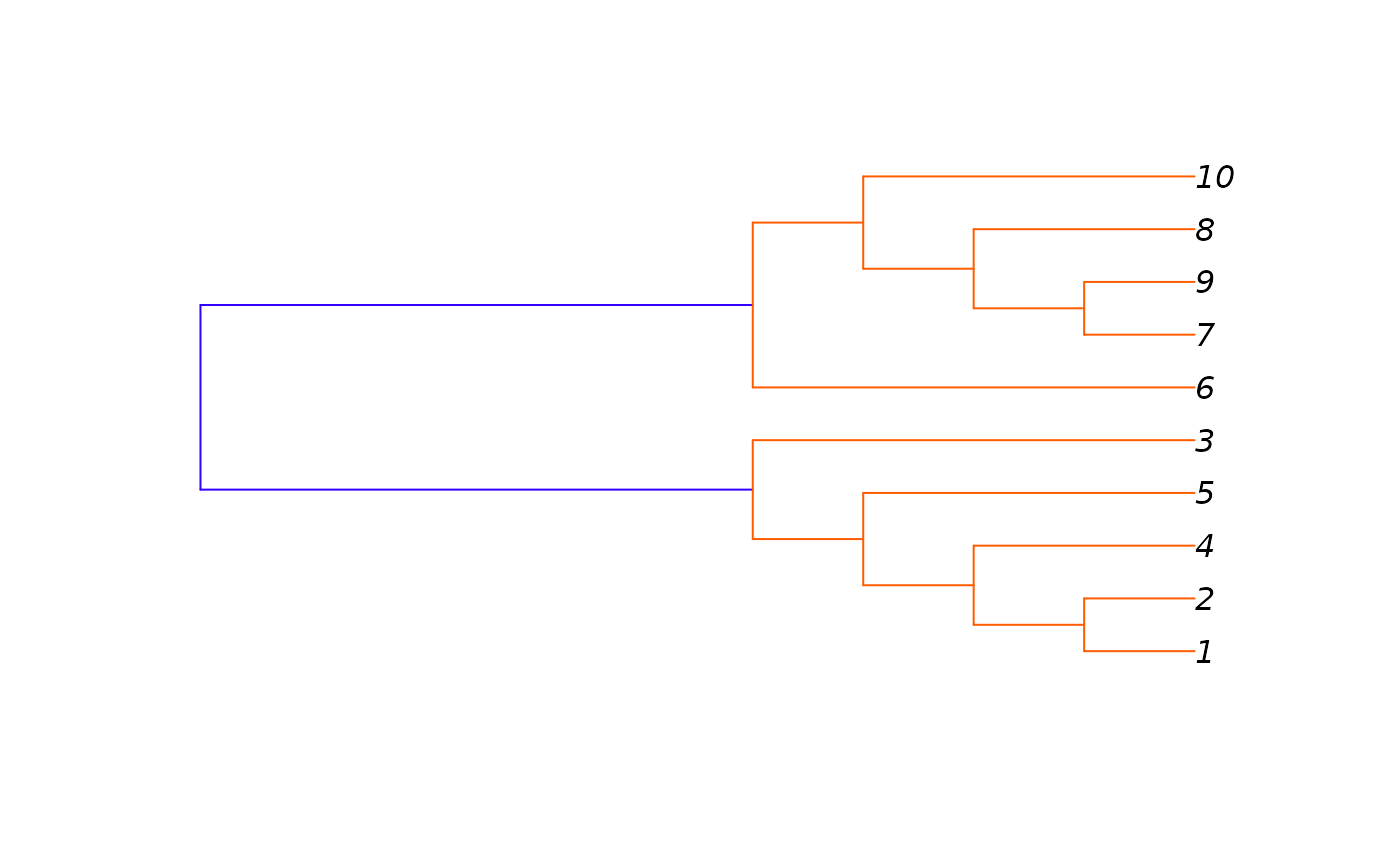Plot a hierarchical random graph as a dendrogram.

## Usage

# S3 method for igraphHRG
plot_dendrogram(x, mode = igraph_opt("dend.plot.type"), ...)

## Arguments

x

An igraphHRG, a hierarchical random graph, as returned by the fit_hrg() function.

mode

Which dendrogram plotting function to use. See details below.

...

Additional arguments to supply to the dendrogram plotting function.

## Value

Returns whatever the return value was from the plotting function, plot.phylo, plot.dendrogram or plot.hclust.

## Details

plot_dendrogram() supports three different plotting functions, selected via the mode argument. By default the plotting function is taken from the dend.plot.type igraph option, and it has for possible values:

• auto Choose automatically between the plotting functions. As plot.phylo is the most sophisticated, that is choosen, whenever the ape package is available. Otherwise plot.hclust is used.

• phylo Use plot.phylo from the ape package.

• hclust Use plot.hclust from the stats package.

• dendrogram Use plot.dendrogram from the stats package.

The different plotting functions take different sets of arguments. When using plot.phylo (mode="phylo"), we have the following syntax:


plot_dendrogram(x, mode="phylo", colbar = rainbow(11, start=0.7,
end=0.1), edge.color = NULL, use.edge.length = FALSE, \dots)


The extra arguments not documented above:

• colbar Color bar for the edges.

• edge.color Edge colors. If NULL, then the colbar argument is used.

• use.edge.length Passed to plot.phylo.

• dots Attitional arguments to pass to plot.phylo.

The syntax for plot.hclust (mode="hclust"):


plot_dendrogram(x, mode="hclust", rect = 0, colbar = rainbow(rect),
hang = 0.01, ann = FALSE, main = "", sub = "", xlab = "",
ylab = "", \dots)


The extra arguments not documented above:

• rect A numeric scalar, the number of groups to mark on the dendrogram. The dendrogram is cut into exactly rect groups and they are marked via the rect.hclust command. Set this to zero if you don't want to mark any groups.

• colbar The colors of the rectangles that mark the vertex groups via the rect argument.

• hang Where to put the leaf nodes, this corresponds to the hang argument of plot.hclust.

• ann Whether to annotate the plot, the ann argument of plot.hclust.

• main The main title of the plot, the main argument of plot.hclust.

• sub The sub-title of the plot, the sub argument of plot.hclust.

• xlab The label on the horizontal axis, passed to plot.hclust.

• ylab The label on the vertical axis, passed to plot.hclust.

• dots Attitional arguments to pass to plot.hclust.

The syntax for plot.dendrogram (mode="dendrogram"):


plot_dendrogram(x, \dots)


The extra arguments are simply passed to as.dendrogram().

## Author

Gabor Csardi csardi.gabor@gmail.com

## Examples


g <- make_full_graph(5) + make_full_graph(5)
hrg <- fit_hrg(g)
plot_dendrogram(hrg)